УДК 004.942

# РЕШЕНИЕ ПРОБЛЕМ ОХРАНЫ ВОЗДУШНОГО БАССЕЙНА В ПРОГРАММНОЙ СРЕДЕ COMSOL MULTIPHYSICS (НА ПРИМЕРЕ ДИФФУЗИИ БИОГАЗА С ПОЛИГОНОВ ТБО И ПО). ИСПОЛЬЗОВАНИЕ COMSOL SCRIPT™

Сауц Артур Валерьевич
ФГБОУ ВПО "Санкт-Петербургский государственный архитектурно-строительный университет"

Аннотация
Статья посвящена моделированию распространения загрязнения атмосферы биогазом, выделяющимся при разложении отходов, в программной среде Comsol Multiphysics. На языке Comsol Script™ написана программа, позволяющая задать начальные и граничные условия, параметры среды, произвести расчёт турбулентной вязкости. Написанная программа является альтернативой недостающего модуля, позволяющего решать в Comsol Multiphysics прикладные задачи, связанные с охраной воздушного бассейна при моделировании рассеивания «холодных» выбросов.

# SOLUTION OF PROBLEMS OF AIR PROTECTION IN THE COMSOL MULTIPHYSICS (FOR EXAMPLE, DIFFUSION OF BIOGAS FROM LANDFILLS). USING COMSOL SCRIPT ™

Sauts Arthur Valerievich
Saint-Petersburg State University of Architecture and Civil engineering

Abstract
The article describes the modeling of the pollution of the atmosphere biogas released during the decomposition of waste in the computer program Comsol Multiphysics. On Comsol Script ™ has written a program that allows specifying the initial and boundary conditions, the parameters of the medium, to calculate the turbulent viscosity. The written program is an alternative to the missing module allowing to Comsol Multiphysics solve applied problems relating to the protection of the air basin modeling dispersion of "cold" emissions.

Библиографическая ссылка на статью:
Сауц А.В. Solution of problems of air protection in the Comsol Multiphysics (for example, diffusion of biogas from landfills). Using Comsol Script ™ // Современная техника и технологии. 2013. № 10 [Электронный ресурс]. URL: http://technology.snauka.ru/2013/10/2412 (дата обращения: 08.02.2019).

The software package Comsol Multiphysics, which implements the numerical solution of equations of mathematical physics, partial (PDE) by finite element/volume, is now widely used to solve problems in various fields of science and technology. The advantages of this package is that it supports a programming language Comsol Script, which allows you to specify non-trivial initial and boundary conditions , the physical parameters of the environment , to work together various modules package Comsol Multiphysics  . In this paper, the language of the Comsol Script written a program using which in Comsol Multiphysics solved urgent task of Applied Ecology – Mathematical modeling of air pollution biogas , outstanding in the decomposition of solid waste and industrial waste in landfills.

Dissipation in the atmospheric surface layer with a passive tracer concentration, mg per m3, described by unsteady turbulent diffusion equation: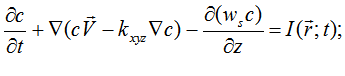(1)

there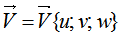is vector velocity, m per sec; ws is sedimentation rate impurities: for light inert gas impurities ws=0 m per sec; for heavy ws = 0,001 m per sec; for aerosols ws = 0,008 m per sec ; kхуz is coefficient of turbulent viscosity in different directions, m2 per sec;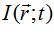is function emissions of impurities in the atmosphere, which is determined by the equation: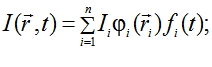(2)

There Ii is function emissions from i-th source; fi (t) and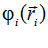are functions describing the mode of operation and location of stationary sources, connected to the radius-vector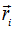and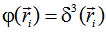. For continuously operating sources fi (t) = 1.

Because the movement of air is a subsonic, in accordance with the approximation of the Boussinesq approximation can be described by a system of equations of the Navier-Stokes equations for an incompressible viscous fluid: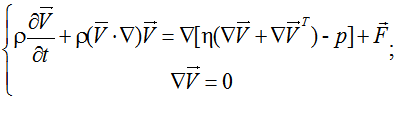(3)

There n is dynamic viscosity, Pa·sec; р is piezometric pressure, Pa;  p is the density of the medium (air), kg per m3;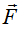is specific force field, N/m3.

Density of air can be found using the equation of state of an ideal gas: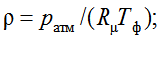(4)

There ратм is atmospheric pressure, Pа; Rm is gas constant, for air Rm = 3,464×10-3 J per kg×K×mol; Тф is background temperature, K.

Kinematic viscosity can be found using the approximation :

h = 6×10-6 + 4×10-8×Тф.                                                                                                     (5)

Turbulent flow structure, which cannot be solved explicitly for the numerical solution of a system of Navier-Stokes equations, are approximated using the subgrid turbulence Smagorinsky model. This approach proposed by Deardorff and developed in the works of Smagorinsky, involves the allocation of the scale, smaller grid, which is approximated by the turbulence . The preference of this model is given to the fact that it takes into account the presence of turbulence, formed a small vortices while flowing wind flow walls of buildings, structures and other obstacles, but the vortices don’t need to approximate the «wall-functions». This approach due to the reduction of the dimension of the matrix equations by a sequence of calculations reduces the requirements to computing resources of a computer.

The scale of small eddies in the Smagorinsky model determined time-measures Dх, Dу, Dz three-dimensional cell: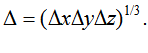(6)

The coefficients of turbulent viscosity is determined using the system: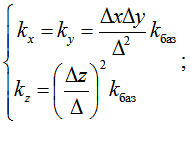(7)

There kбаз is basic coefficient of turbulent viscosity, and contempt of the temperature gradient low altitude and temperature of the discharged impurities determined the approximate equation:(8)

There kф is background coefficient of turbulent viscosity, kф = 1-15 м2/с; ε = 0,1-0,4; Def (x; y; z) is function of deformation or dissipation defined by the equation: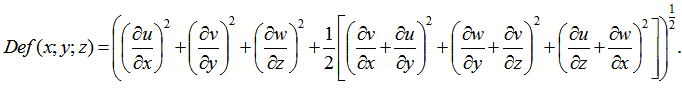At the upstream boundary of the computational domain profile of wind speed is given by Karman. Since wind flow can be directed at an angle of the input boundary of the computational domain, the velocity components of the system are given by: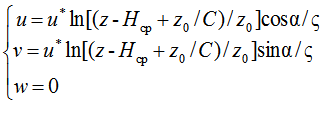(9)

There u* is dynamic speed, m per sec; z0 is argument of a roughness;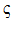is Karman`s constant,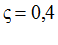; Нср is the average height of wind barriers; С is coefficient of resistance;
α is the angle between the direction of the wind on the input in the computational domain boundary and the x-axis, radian.

At the downstream boundary of the computational domain is given the type of border Outlet, the boundary condition Normal flow / zero pressure:

р = 0.                                                                                                          (10)

On the surfaces of buildings and structures, landfill, land for the components of the wind speed is given by the condition of impermeability and adhesion: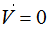(11)

If the streamlined surface is the area where is blowing (suction) air velocity components uв, vв, wв, then the area is given by the boundary condition Moving /Leaking wall:

и = ив; v = vв; w = wв.                                                                                          (12)

On the lateral boundaries of the computational domain is specified Symmetry boundary, meaning the absence of leaks and tangential stresses: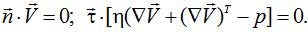(13)

Thereand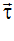is normal and tangential unit vector to the boundary surface, unit vectordirected outward from the boundary region.

At presence on a surface of the polygon of ground insulation filling and absence of gas drainage wells circuit equations emissions and diffusion components of biogas is produced with the help of the derived equations :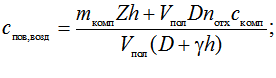(14)

There D is diffusivity of landfilling (insulating the outside waste), m2 per sec; h is thickness of landfilling, m; nотх is porosity of waste, nотх = 0,33; γ is permeation coefficient, for gases γ = 0,01-0,015 m per sec; спов,возд is the concentration of component biogas at the upper side of air filling, mg per m3.

On the surface of the landfill to calculate the concentration of a component of biogas in the absence of gas drainage wells getsand sets boundary condition:

c = спов,возд.                                                                                                 (15)

If the emission biogas through gas drainage wells or surface landfill, which has no insulating filling, the consideration of the effect of each of them by using boundary condition Flux: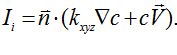(16)

On impervious surfaces flow of impurities (Ii = 0) is given by boundary condition Isolation/Symmetry: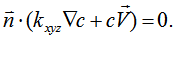(17)

On the other boundaries of the computational domain is given by the boundary condition Convective flux:(18)

When determining the scale of small eddies in the model of the radical work of Smagorinsky DхDуDz can be interpreted as the volume of the rectangular cell using MCS . When using the finite element method with the most common type of cells – tetrahedral most famous software packages such as Ansys CFX, Fluent, Flow Vision, etc. In this case, the volume of each cell is calculated by means of numerical methods. To determine the scale, the following simpler approach. The maximum linear dimension of the finite element and should be much smaller than the calculated area, the growth rate of the element responsible for the degree of condensation, taken strictly to 1. These options allow you to get a thick, almost uniform grid consisting of a set of tetrahedral that are close to correct. Then, in our case for small scale vortices can be approximated as the cube root of the volume of the tetrahedron: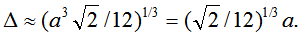(19)
Then, using the approximation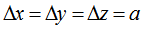, we obtain: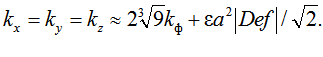(20)

In our case, made ​​a = 4 m. At this density grid in  by the method of successive approximations for calculating the concentrations of methane emitted at the site ” Centralny” in Volgograd , the optimal values ​​of kф = 4 m2/s ; ε = 0,2. This approach eliminates the calculation of sub-grid scale using tetrahedral finite element mesh, thereby simplifying the numerical (software) implementation of the turbulence model and reduces the requirements for compute-intensive computer.

From a practical point of view is suitable stationary statement of the problem. In this case, a method of determining where the problem is solved on a large time interval that the process leads to stationary. Implicit scheme allows to get rid of restrictions to the size of the time step. Stationary statement of the problem significantly reduces the number of iterations, and the consumption of computing resources of a computer.

The solution of the discrete analogues of the differential equations made by bi-conjugate gradients with the stabilization of the solution. The whole program code is more than a dozen pages, so in an article for the sake of brevity, it shows only the main conclusions:

% COMSOL Multiphysics Model M-file

% Generated by COMSOL 3.4 (COMSOL 3.4.0, \$Date: 2007/10/03 17:02:19 \$)

flclear fem

% COMSOL version

clear vrsn

vrsn.name = ‘COMSOL 3.4′;

vrsn.ext = ‘a’;

vrsn.major = 0;

vrsn.build = 603;

vrsn.rcs = ‘\$Name: \$’;

vrsn.date = ‘\$Date: 2007/10/03 17:02:19 \$’;

fem.version = vrsn;

% Constants

% Constants
fem.const = {‘W_s’,’0′, …
‘k_f’,’4′, …
‘epsilon’,’0.2′, …
‘Mcomp’,’5′, …
‘h_iz’,’0.2′, …
‘Vpol’,’100000′, …
‘Diffusiv’,’3.4e-5′, …
‘gamma’,’0.001′, …
‘Ccomp’,’50′, …
‘Pamb’,’1e5′, …
‘T_amb’,’25′, …
‘Udin’,’3′, …
‘Hsr’,’10′, …
‘Z0′,’0.2′, …
‘C’,’0.0115′, …
‘alpha’,’0′, …
‘a’,’4′};

% Geometry

% (Default values are not included)

% Application mode 1
clear appl
appl.mode.class = ‘FlNavierStokes’;
appl.shape = {‘shlag(1,”u”)’,'shlag(1,”v”)’,'shlag(1,”w”)’,'shlag(1,”p”)’};
appl.gporder = 2;
appl.cporder = 1;
appl.assignsuffix = ‘_ns’;
clear prop
prop.analysis=’static’;
appl.prop = prop;
clear pnt
pnt.p0 = {};
pnt.name = {};
pnt.pnton = {};
pnt.ind = [];
appl.pnt = pnt;
clear bnd
bnd.U0out = {};
bnd.v0 = {};
bnd.opentype = {};
bnd.u0 = {};
bnd.Fbnd = {};
bnd.p0 = {};
bnd.type = {};
bnd.velType = {};
bnd.walltype = {};
bnd.w0 = {};
bnd.inttype = {};
bnd.vw = {};
bnd.U0in = {};
bnd.ww = {};
bnd.uw = {};
bnd.name = {};
bnd.outtype = {};
bnd.f0 = {};
bnd.intype = {};
bnd.stresstype = {};
bnd.ind = [];
appl.bnd = bnd;
clear equ
equ.eta = ’6e-6+T_amb*4e-8′;
equ.name = ‘physical parameters of the atmosphere in the zone of landfill’;
equ.rho = ‘Pamb*3.464e-3/T_amb’;
equ.ind = [];
appl.equ = equ;
fem.appl{1} = appl;

% Application mode 2
clear appl
appl.mode.class = ‘FlConvDiff’;
appl.assignsuffix = ‘_cd’;
clear prop
prop.analysis=’static’;
clear weakconstr
weakconstr.value = ‘off’;
weakconstr.dim = {‘lm5′};
prop.weakconstr = weakconstr;
appl.prop = prop;
clear bnd
bnd.d = {};
bnd.Dbnd = {};
bnd.c0 = {};
bnd.name = {};
bnd.N = {};
bnd.type = {};
bnd.ind = [];
appl.bnd = bnd;
clear equ
equ.D = ’4.160167646*k_f+0.707106781*epsilon*(a^2)*((ux)^2+(vy)^2+(wz)^2+0.5*((vx+uy)^2+(wy+vz)^2+(uz+wx)^2))^0.5′;
equ.w = ‘w-W_s’;
equ.v = ‘v’;
equ.u = ‘u’;
equ.name = ‘physical parameters of the atmosphere in the zone of landfill’;
equ.ind = [];
appl.equ = equ;
fem.appl{2} = appl;
fem.sdim = {‘x’,'y’,'z’};
fem.frame = {‘ref’};
fem.border = 1;
clear units;
units.basesystem = ‘SI’;
fem.units = units;

% Scalar expressions
fem.expr = {‘profil_u’,’2.5*Udin*log((z-Hsr+Z0/C)/Z0)*cos(alpha)’, …
‘profil_v’,’2.5*Udin*log((z-Hsr+Z0/C)/Z0)*sin(alpha)’, …
‘Cpov’,'(Mcomp*z_iz*h_izol/Vpol+0.33*Diffusiv*Ccomp)/(Diffusiv+gamma*h_iz)’, …
‘Mud’,'Mcomp*z/Vpol’};

% Descriptions
clear descr
descr.expr= {‘Mud’,'emission component of biogas from the landfill area, mg / (kv.m.sek) (boundary condition of the 2nd kind is used in the absence of landfilling)’,'Cpov’,'concentration of the component of biogas at the landfill surface (boundary condition of the 2nd kind is used in the absence of landfilling)’,'profil_v’,'y-oic wind component on the input boundary of the computational domain’,'profil_u’,'x-oic wind component on the input boundary of the computational domain’};
fem.descr = descr;

% Descriptions
descr = fem.descr;
descr.const= {‘h_iz’,'thickness of the insulating filling (default made ??0.2 m)’,'Pamb’,'atmospheric pressure Pa’,'Vpol’,'volume occupied by the solid waste landfill or softwareб cubic m’,'Hsr’,'average height of wind barriers, m’,'Diffusiv’,'diffusivity of the soil from which the filling,square m/seс’,'a’,'maximum line size tetraedricheskogo finite element, m’,'C’,'roughness parameter’,'k_f’,'background coefficient eddy viscosity Smagorinsky model, square m/sec (default is assumed to be 4)’,'Mcomp’,'emission component of the biogas emitted from around the landfill or software mg/sec’,'Udin’,'dynamic speed, m/s’,'Z0′,’resistance coefficient, m’,'W_s’,'settling velocity of the impurities in the atmosphere for light inert gas impurities 0; Heavy 0.001, 0.008 for aerosols m/sec’,'Ccomp’,'the concentration of the components in the biogas at the time of its release in the body of the landfill, mg/cubic m’,'gamma’,'coefficient of gas seepage through the soil, m/sec (default is 0.001)’,'alpha’,'angle between the direction of the wind at the entrance to the settlement area boundary and the x axis, radian’,'epsilon’,'constant in the Smagorinsky model (the default is assumed to be 0.2)’,'T_amb’,'background air temperature, K’};
fem.descr = descr;

% ODE Settings
clear ode
clear units;
units.basesystem = ‘SI’;
ode.units = units;
fem.ode=ode;

% Multiphysics
fem=multiphysics(fem);

The settlements were made on the computer AMD FX™-4100 Quard-Core Processor, RAM 4 Gb, 3,2 GHz, OS MS Windows 7 Ultimate SP1. The calculation time for landfill «Centralny» was about 30 hours. The results of calculations are shown in figures 1-2 (unit mol per m3 treated as mg per m3).Figure 1 – The field of wind speed, m per sec, at the height of 1.8 m above the top of the landfill site landfill PTO-3 «Novosyolki» 26.08.2001Figure 2 – Field concentrations of methane, mg per m3, at the height of 1.8 m above the top of the landfill site PTO-3 «Novosyolki» 26.08.2001

References
1. COMSOL Script User`s Guide. Version 1.1. – April, 2006. – URL: http://www.mscs.dal.ca/cluster/manuals/comsol/scriptug.pdf (date of access 15.11.2013).
2. Теверовский, Е.Н., Перенос аэрозольных частиц турбулентными потоками / Е.Н.Теверовский, Е.С.Дмитриев / – М.: Энергоатомиздат, 1988. – 160 с.
3. Егоров, В.И. Применение ЭВМ для решения задач теплопроводности. Учебное пособие / В.И.Егоров. – СПб: СПб ГУ ИТМО, 2006. – 77 с.
4. Deardorff, J.W. A three-dimensional numerical investigation of the idealized planetary boundary layer // Geophysics. Fluid Dynamic, 1970. – v. 1. – p. 377-410.
5. Сауц, А.В. Граничные условия численного моделирования рассеивания биогаза над полигоном / А.В.Сауц / Инженерно-экологические системы: материалы Междунар. конгресса, посвящённого 180-летию СПБГАСУ. – СПб: СПБГАСУ, 2012. – С. 155-158.
6. Sauts, A.V. Modeling of atmospheric pollution biogas from landfills with by means of solutions of the equations of turbulent diffusion and the Navier-Stokes equations / A.V.Sauts / Modern scientific researches and innovations. Electronic journal. – March, 2013. – URL: http://web.snauka.ru/en/issues/2013/03/22980 (date of access 15.11.2013).

Все статьи автора «artursauc»

© Если вы обнаружили нарушение авторских или смежных прав, пожалуйста, незамедлительно сообщите нам об этом по электронной почте или через форму обратной связи.